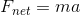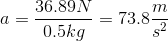# MCAT Physical : Buoyancy and Displacement

## Example Questions

### Example Question #1 : Buoyancy And Displacement

Use the following information to answer questions 1-6:

The circulatory system of humans is a closed system consisting of a pump that moves blood throughout the body through arteries, capillaries, and veins. The capillaries are small and thin, allowing blood to easily perfuse the organ systems. Being a closed system, we can model the human circulatory system like an electrical circuit, making modifications for the use of a fluid rather than electrons. The heart acts as the primary force for movement of the fluid, the fluid moves through arteries and veins, and resistance to blood flow occurs depending on perfusion rates.

To model the behavior of fluids in the circulatory system, we can modify Ohm’s law of V = IR to ∆P = FR where ∆P is the change in pressure (mmHg), F is the rate of flow (ml/min), and R is resistance to flow (mm Hg/ml/min). Resistance to fluid flow in a tube is described by Poiseuille’s law: R = 8hl/πr4 where l is the length of the tube, h is the viscosity of the fluid, and r is the radius of the tube. Viscosity of blood is higher than water due to the presence of blood cells such as erythrocytes, leukocytes, and thrombocytes.

The above equations hold true for smooth, laminar flow. Deviations occur, however, when turbulent flow is present. Turbulent flow can be described as nonlinear or tumultuous, with whirling, glugging or otherwise unpredictable flow rates. Turbulence can occur when the anatomy of the tube deviates, for example during sharp bends or compressions. We can also get turbulent flow when the velocity exceeds critical velocity vc, defined below.

vc = NRh/ρD

NR is Reynold’s constant, h is the viscosity of the fluid, ρ is the density of the fluid, and D is the diameter of the tube. The density of blood is measured to be 1060 kg/m3.

Another key feature of the circulatory system is that it is set up such that the organ systems act in parallel rather than in series. This allows the body to modify how much blood is flowing to each organ system, which would not be possible under a serial construction. This setup is represented in Figure 1.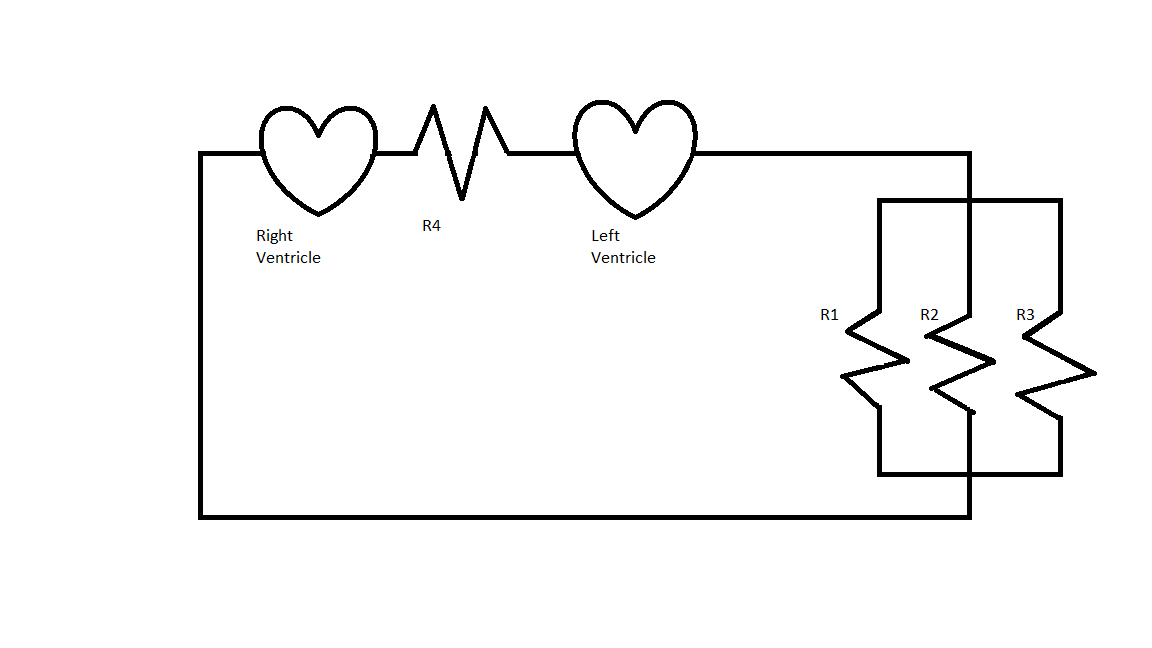A red blood cell floating in the body weighs 27 picograms and has a volume of 40.0 * 10-12cm3. What is the buoyant force on the red blood cell?

2.7 * 10-10N

2.7N

4 * 10-7N

4 * 10-13N

4 * 10-13N

Explanation:

To answer this question, we use Fb = Vgρ, where V is volume, g is gravity, and ρ is the density of the fluid.

In this case we can use the density of blood, since it is given in the passage as 1060kg/m3. Since the density is in cubic meters, we have to make sure that the volume is also converted to cubic meters.

(4.0 * 10-11)x (1 * 10-6m/ 1cm) = 4 * 10-17m3

Fb = (4.0 x 10-17m3) (10 m/s2) (1.06 x 103kg/m3) = 4 * 10-13N

### Example Question #2 : Buoyancy And Displacement

Two identical balloons, balloon A and balloon B, are filled with gas A and gas B, respectively. In which described scenario will the balloon rise the fastest?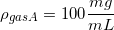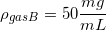Balloon A at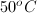All scenarios describe equal rising rates

Balloon A at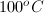Balloon B atBalloon B atBalloon B atExplanation:

As temperature increases, density decreases.

In a free body diagram of each balloon, the only forces acting in the vertical direction are gravity and buoyant force.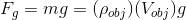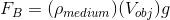These forces act in opposite directions, with the buoyant force pulling up and gravity pulling down.

As gas B is heated to the higher temperature, the density decreases. Gas B already has a lower density than gas A; heating it simply intensifies this discrepancy. As the density decreases, the force of gravity on the balloon decreases as well, allowing a greater net force in the upward direction due to the buoyant force.

The net upward force experienced by balloon B at the highest temperature will be greater than any of the other scenarios listed, resulting in the greatest upward acceleration.

### Example Question #3 : Buoyancy And Displacement

An irregular solid has a mass of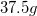on a laboratory balance. It is suspended by a thread from a spring scale and fully immersed in water. The spring scale reads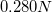. What is the buoyant force on the solid?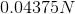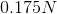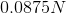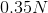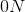Explanation:

The difference between the mass as measured in air and the mass as measured when it is suspended in water is the mass of the displaced water, easily reduced to the volume of the solid.

First calculate the weight of the solid in air.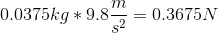In water, it weighs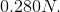The difference between these values is the buoyant force acting on the solid.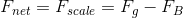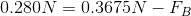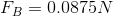Recall that buoyant force is equal to the weight of water displaced by the object.

### Example Question #4 : Buoyancy And Displacement

Two students in a physics class are conducting an experiment to see how different objects displace water in a container. Student A places a lead spherical marble with a radius of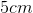in the container. Student B places a considerably lighter solid glass sphere in the container, with twice the volume of the lead marble. Which of the following statements is true?

I. The amount of displaced water depends on the mass of a fully immersed object

II. The amount of water displaced depends on the volume of a fully immersed object

III. Student A's object will make the water level rise more than Student B's

IV. Student B's object will make the water level rise more than Student A's

V. From the information given, one cannot determine which object would displace more water

V only

II only

II and IV

I and III

IV only

II and IV

Explanation:

This question relates directly to Archimedes' principle considering buoyancy. The take home points of this question are given in the answers:

1) The more volume an object occupies, the more water it can displace.
2) The mass of an object has no relevance to the amount of water displaced as long the object is fully immersed.

From the second of these two points, we can eliminate statement I since the amount of water displaced is independent of mass. We can also eliminate statements III and V, since the marble with greater volume will displace a greater volume of water. This leaves the correct statements: II and IV.

### Example Question #5 : Buoyancy And Displacement

A 100g block of copper, a 100g block of aluminum, and a 100g sphere of gold are placed into a large tub of water. Which object expereiences the greatest buoyant force?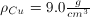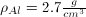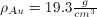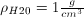Gold

Copper

Aluminum

There is not enough information, without knowing the depth of each object

Two or more experience the same buoyant force

Aluminum

Explanation:

Buoyant force depends only on the density of the fluid, and the volume of fluid displaced, according to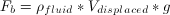. Since each object has a density greater than that of water, they will all be completely submerged. So, the volume of fluid displaced equals the volume of the object in each case, meaning that whichever object has the largest volume will experience the greatest buoyant force.

For copper: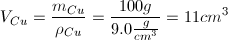For aluminum: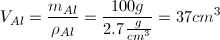For gold: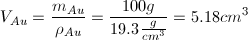Since aluminum has the largest volume, and the largest volume of water displaced, it experiences the greatest buoyant force.

### Example Question #6 : Buoyancy And Displacement

When a copper sphere is suspended from a spring scale in air, the scale reads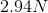. Assuming the density of copper is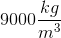, what will the scale read if the sphere is lowered (completely submerged) in a large beaker of water?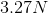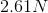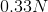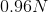Explanation:

We need to find the buoyant force on the sphere to see how much the scale reading changes.

Buoyant force is given by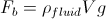, where V is the volume displaced by the sphere.

We need to find this volume, which also equals the sphere's volume. Given the sphere's mass (m = F/g = 2.94/9.8 = 0.30kg) and density, we can find volume with either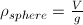or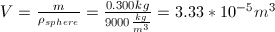.

Plugging this volume into the buoyant force equation, together with the density of water (1000 kg/m3) gives the following.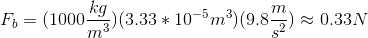So now the upwards force provided by the scale is reduced by 0.33N.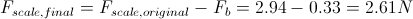### Example Question #7 : Buoyancy And Displacement

A soccer ball with a mass of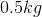and diameter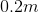is held under water. What is the acceleration of the ball the instant it is released?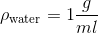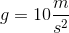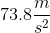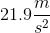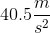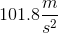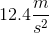Explanation:

To calculate the ball's instantaneous acceleration, we need to calculate the net force on the ball. We can neglect the resistance due to water since we are calculating the net force the moment the ball is released, before it has begun to move. Therefore, there are only two forces on the ball: gravitational force and buoyant force.

Force of gravity: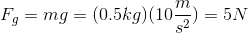The bouyant force is simply the force of water that is displaced by the ball: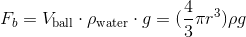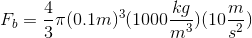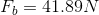Now we can calculate the net force on the ball: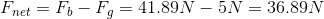We can simply use Newton's second law to calculate the acceleration of the ball: